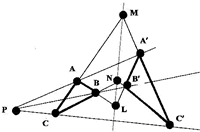# Geometry Vol 2These notes include a first course on Projective Geometry, using Linear Algebra.

Projective points are defined as one dimensional subspaces of 3 dimensional space and projective lines are two dimensional subspaces.

Among other applications, this gives a much more efficient way of proving those theorems in Euclidean Geometry that deal only with points lying on lines, such as Desargues Theorem and Pappus Theorem and Cross ratio theorems.

Other chapters deal with isometries, and symmetry, both in two and three dimensions

Finally there is a discussion of ruler and compass constructions, including constructions of the foci and tangents for a given parabola or ellipse.

The impossibility of trisecting any given angle by ruler and compass is proved

While a parabola or ellipse cannot be drawn using ruler and compass, several methods are shown for constructing any finite number of points on an ellipse, when the foci are given.

And an ellipse can be drawn using string and pins.

There is some discussion of string and pins constructibility, including the fact that any angle can be trisected by a string and pins construction, but this is not the case if we want to divide any given angle into five equal parts.

[Please note that all links are to Adobe .pdf documents. They will open in a separate browser window.]